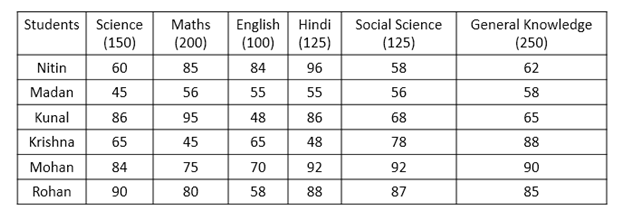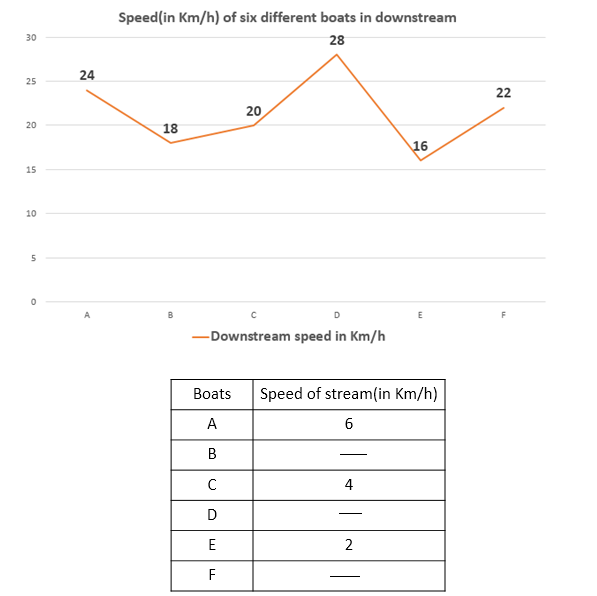# Quantitative Aptitude Questions (Data Interpretation) for SBI Clerk / IDBI Executive 2018 Day-66

Dear Readers, SBI is conducting Online preliminary Examination for the recruitment of Clerical Cadre. preliminary Examination of SBI Clerk was scheduled from June/July 2018. To enrich your preparation here we have providing new series of Data Interpretation – Quantitative Aptitude Questions. Candidates those who are appearing in SBI Clerk Prelims and IDBI Executive Exams can practice these Quantitative Aptitude average questions daily and make your preparation effective.

Click “Start Quiz” to attend these Questions and view Solutions

Directions (Q. 1 – 5): Study the following information carefully and answer the questions given below:

The following table represents percentage of marks obtained by six different students in six different subjects and maximum marks of each subject.1. Marks obtained by Madan in Hindi is what percent of marks obtained by Krishna in General Knowledge?

a) 35.62%

b) 31.25%

c) 21.22%

d) 16.25%

e) None of these

2. Find the total marks obtained by all the students in Science.

a) 465

b) 645

c) 654

d) 456

e) None of these

3. Find the overall percentage of marks obtained by Kunal in all the subjects.

a) 45%

b) 76%

c) 74%

d) 67%

e) None of these

4. Find the respective ratio of marks obtained by Nitin in Science, Maths and English together and marks obtained by Mohan in Hindi, Social Science and General Knowledge together.

a) 455 : 344

b) 344 : 455

c) 341 : 435

d) 134 : 344

e) None of these

5. Find the difference between total marks obtained by Krishna and Rohan in all the subjects together.

a) 135

b) 154.25

c) 145.5

d) 125.75

e) None of these

Directions (Q. 6 – 10): Study the following information carefully and answer the questions given below:

The following line graph represents the downstream speeds of six different boats.6. Find the sum of the time taken by A to travel 36 km upstream and the time taken by E to travel 48 km upstream.

a) 3 hours

b) 5 hours

c) 7 hours

d) 2 hours

e) None of these

7. If boat D goes 66 km in upstream in the same time as 84 km downstream, than find the speed of Stream?

a) 12 km/hr

b) 8 km/hr

c) 6 km/hr

d) 3 km/hr

e) None of these

8. If boat F can go 48 km in 3 hours in still water, find how much distance it can go upstream in 3 hours?

a) 33 km

b) 30 km

c) 25 km

d) 24 km

e) None of these

9. Find the difference between the time taken by boat C to travel 80 km downstream and time taken by boat E to travel 84 km upstream.

a) 3 hours

b) 4 hours

c) 2 hours

d) 5 hours

e) None of these

10. If boat B can go 50 km upstream in 5 hours, find the speed of the stream.

a) 2 km/hr

b) 6 km/hr

c) 4 km/hr

d) 3 km/hr

e) None of these

Directions (Q. 1 – 5):

Marks obtained by Madan in Hindi = 55/100 x 125 = 68.75

Marks obtained by Krishna in General Knowledge = 88/100 x 250 = 220

Required percentage = 68.75/220 x 100 = 31.25%

Total marks obtained by all the students in Science

= (60 + 45 + 86 + 65 + 84 + 90) * 150/100

= 430 x 150/100 = 645

Marks obtained by Kunal in,

Science = (86/100) x 150 = 129

Maths = (95/100) x 200 = 190

English = (48/100) x 100 = 48

Hindi = (86/100) x 125 = 107.5

Social Science = (68/100) x 125 = 85

General Knowledge = (65/100) x 250 = 162.5

Overall percentage

= [(129 + 190 + 48 + 107.5 + 85 + 162.5)/(150 + 200 + 100 + 125 + 125 + 250)] x 100

= 722/950 x 100 = 76%

Marks obtained by Nitin in:

Science = (60/100) x 150 = 90

Maths = (85/100) x 200 = 170

English = (84/100) x 100 = 84

Marks obtained by Mohan in:

Hindi = (92/100) x 125 = 115

Social Science = (92/100) x 125 = 115

General Knowledge = (90/100) x 250 = 225

Required ratio = (90 + 170 + 84) : (115 + 115 + 225) = 344 : 455

Marks obtained by Krishna in all the subjects

= (65/100) x 150 + (45/100) x 200 + (65/100) x 100 + (48/100) x 125 + (78/100) x 125 + (88/100) x 250

= 97.5 + 90 + 65 + 60 + 97.5 + 220

= 630

Marks obtained by Rohan in all the subjects

= (90/100) x 150 + (80/100) x 200 + (58/100) x 100 + (88/100) x 125 + (87/100) x 125 + (85/100) x 250

= 135 + 160 + 58 + 110 + 108.75 + 212.5

= 784.25

Required difference = 784.25 – 630 = 154.25

Directions (Q. 6 – 10):

For boat A:

Speed of the boat in still water = 24 – 6 = 18km/h

Upstream speed = 18 – 6 = 12km/h

For boat E:

Speed of the boat in still water = 16 – 2 = 14km/h

Upstream speed = 14 – 2 = 12km/h

Required sum of the time = 36/12 + 48/12 = 3 + 4 = 7 hours.

Let speed of boat D in the stream = y km/hr

Time taken by D to go 84 km downstream = 84/28 = 3 hours

Speed of upstream = 66/3 = 22 km/hr

Speed of stream(y) = ½(speed of downstream – speed of upstream)

= > ½(28 – 22)

=> y = 3 km/hr

Let speed in still water = x km/hr

Speed of the stream = y km/hr

x = 48/3 = 16

And we have,

x + y = 22

y = 22 – x = 22 – 16 = 6 km/hr

Speed of upstream = 16 – 6) = 10 km/hr

Required distance = 10 * 3 = 30 km

Time taken by boat C to travel to travel 80 km downstream = 80/20 = 4 hours

Downstream speed of boat E = 16km/hr

Speed of stream = 2km/hr

Upstream speed = 16 – 2 – 2 = 12 km/hr

Time taken by boat E to travel 84 km upstream = 84/12 = 7 hours

Required difference = 7 – 4 = 3 hours.

Let speed of upstream be x km/hr and the speed downstream be y km/hr

Speed of upstream(x) = 50/5 = 10 km/hr

Speed of Downstream(y) = 18 km/hr

Speed of stream = ½(x – y) = ½(18 – 10)

= > 4 km/hr

Daily Practice Test Schedule | Good Luck

 Topic Daily Publishing Time Daily News Papers & Editorials 8.00 AM Current Affairs Quiz 9.00 AM Logical Reasoning 10.00 AM Quantitative Aptitude “20-20” 11.00 AM Vocabulary (Based on The Hindu) 12.00 PM Static GK Quiz 1.00 PM English Language “20-20” 2.00 PM Banking Awareness Quiz 3.00 PM Reasoning Puzzles & Seating 4.00 PM Daily Current Affairs Updates 5.00 PM Data Interpretation / Application Sums (Topic Wise) 6.00 PM Reasoning Ability “20-20” 7.00 PM English Language (New Pattern Questions) 8.00 PM General / Financial Awareness Quiz 9.00 PM